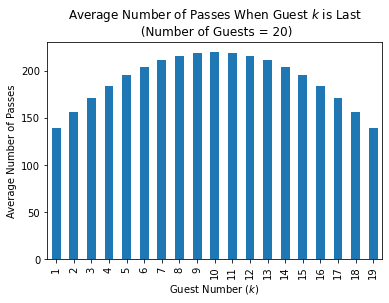Cranberry Sauce Riddler

Prompt:

To celebrate Thanksgiving, you and 19 of your family members are seated at a circular table (socially distanced, of course). Everyone at the table would like a helping of cranberry sauce, which happens to be in front of you at the moment.

Instead of passing the sauce around in a circle, you pass it randomly to the person seated directly to your left or to your right. They then do the same, passing it randomly either to the person to their left or right. This continues until everyone has, at some point, received the cranberry sauce.

Of the 20 people in the circle, who has the greatest chance of being the last to receive the cranberry sauce?

In :
# math
import numpy as np
from math import floor, ceil
import networkx as nx
from collections import OrderedDict

# data manipulation
import pandas as pd

# plotting
import matplotlib.pyplot as plt
import seaborn as sns
import matplotlib.ticker as mtick
from networkx.drawing.nx_agraph import graphviz_layout


## Auxilliary Markov Process¶

The functions in this section are used to provide terminal properties for a drunkard's walk on a finite interval. We use this in the main cranberry passing Markov process to specify the probability that the next guest to receive the cranberry sauce will be next to the person holding the cranberry sauce, or will be the person sitting next to the furthest person who has already been served. In addition, we also calculate the number of passes that will be made in each case.

In :
def drunkard_walk_transition_matrix(n_interval):
# P is the transition matrix
P = np.zeros((n_interval + 2, n_interval + 2))
P[0, 0] = 1
P[n_interval+1, n_interval+1] = 1
for i in range(1, n_interval + 1):
P[i, i-1] = 0.5
P[i, i+1] = 0.5

return P

In :
def drunkard_walk_exit_distro(n_interval):
'''
Compute the probability that a random walk exits an interval of length
n from the left or right, conditioned on beginning at the left-most end of the
interval.

Input:
n_interal: length of interval
max_iter : maximum number of iterations to use

Returns:
(p_near, p_far): where p_near = probability of left-exit, and
p_far = probability of right-exit.
'''

if n_interval == 1:
return 0.5, 0.5

# P is the drunkard's walk transition matrix
P = drunkard_walk_transition_matrix(n_interval)

# Put P in canonical form
n_transient = 2
idxs = list(range(1, n_interval + 1)) + [0, n_interval + 1]
P = P[idxs, :]
P = P[:, idxs]

# Transient submatrix Q and transition to absorbing matrix R
n_absorbing = 2
Q = P[:-n_absorbing, :-n_absorbing]
R = P[:-n_absorbing, -n_absorbing:]
N_inv = np.eye(n_interval) - Q

# Solve for B, whose rows represent absorption probabilities
# starting from a given transient state, and columns represent
# probability of absorption into a specific absorbing state.
# see e.g.
#    https://en.wikipedia.org/wiki/Absorbing_Markov_chain#Absorbing_probabilities
B = np.linalg.solve(N_inv, R)

# We're only interested in absorption from the first state into
# each of the absorbing states.
p_left = B[0, 0]
p_right = B[0, 1]

return p_left, p_right

In :
def drunkard_walk_avg_exit_time_each_side(n_interval):

P = drunkard_walk_transition_matrix(n_interval)

# re-order to put P in "standard form" with absorbing states at
# the end
idxs = list(range(1, n_interval + 1)) + [0, n_interval + 1]
P = P[idxs, :]
P = P[:, idxs]

# Important matrices
Q = P.copy()[:-2, :-2]
R = P.copy()[:n_interval, -2:]
#N = np.linalg.inv(np.eye(n_interval) - Q)
N_inv = np.eye(n_interval) - Q

# Compute B = N*R = (I - Q)^-1 * R
B = np.linalg.solve(np.eye(n_interval) - Q, R)

# compute quantities from B for left/right absorbing states
b_left = B[:, 0]
b_right = B[:, 1]
D_left = np.diag(b_left)
D_right = np.diag(b_right)

# Use theorem 1 from:
#   https://doi.org/10.1016/j.spl.2019.04.001
#   https://www.researchgate.net/publication/332384778_Applications_of_the_fundamental_matrix_to_mean_absorption_and_conditional_mean_absorption_problems
# Using this theorem, we get alpha, the mean number of steps from
# any starting state as follows:
#  alpha_left = D_left^{-1} * N * D_left * xi
#  alpha_right = D_right^{-1} * N * D_right * xi
# where xi is a vector of ones. we're only interested in the first entry of
# alpha_left and alpha_right.
xi = np.ones(n_interval)

# compute mu_left.
xi_left = np.dot(D_left, xi)
alpha_left = np.linalg.solve(N_inv, xi_left)
alpha_left = np.linalg.solve(D_left, alpha_left)
mu_left = alpha_left

# compute mu_right.
xi_right = np.dot(D_right, xi)
alpha_right = np.linalg.solve(N_inv, xi_right)
alpha_right = np.linalg.solve(D_right, alpha_right)
mu_right = alpha_right

return mu_left, mu_right

In :
def drunkard_walk_avg_exit_time(n_interval):
p_left, p_right = drunkard_walk_exit_distro(n_interval)
mu_left, mu_right = drunkard_walk_avg_exit_time_each_side(n_interval)

return p_left * mu_left + p_right * mu_right


## Main Cranberry Passing Markov Process¶

In this process, we are only interested in tracking the "boundary" of the guests who have already been served. States of this system are either

1. a tuple of the form (most_recently_served, interval_served, n_served)
2. an integer representing the last guest to be served.

Transition probabilities in this Markov process are governed by a drunkard's walk on interval_served (i.e. the auxiliary walk indicated above), and indicate whether the nearest unserved guest receives the sauce next, or whether the furthest unserved guest is served next.

Each step in this process represents one new guest being served, and the number of steps in this process does not indicate the number of cranberry sauce passes. To compute the average number of passes requies a bit of work using the number of transitions in the drunkard's walk.

In :
def table_dist(i, j, n_guests):
'''
Compute number of spaces between position i and j around a table
with n_guests

Input:
i, j: position indices for two guests to be compared
n_guests: the number of guests at the table

'''
diff = abs(i - j)

if diff <= floor(n_guests / 2):
return diff
else:
return n_guests - diff

In :
def receiver_states(state, n_guests):
'''
Generate the states which can be reached from the current state.

Input:
state: either a tuple of the form (current_pos, interval, n_served)
or an integer representing a terminal state
n_guests: the number of guests at the table

Return:
receptive_states: either a list of the possible transition states,
if state is not terminal, and None if state is terminal (!!)
'''

if state == (0, (0, 0), 1):
receptive_states = [(n_guests-1, (n_guests-1, 0), 2), (1, (0, 1), 2)]

elif type(state) == tuple:
i, j = state
n_served = state

if n_served == n_guests - 1:
if [i, j] == [0, n_guests - 2]:
receptive_states = [n_guests - 1]
elif [i, j] == [n_guests - 2, 0]:
receptive_states = 
else:
receptive_states = [(i + j) // 2]

else:
i_left  = (i - 1) % n_guests
j_right = (j + 1) % n_guests
receptive_states = [(i_left,  (i_left,  j), n_served + 1),
(j_right, (i, j_right), n_served + 1)]
else:
receptive_states = None

return receptive_states

In :
def generate_states(n_guests):
'''
Generate the transition states for Cranberry passing in sorted order.

Input:
n_guests: number of guests around the table

Return:
transition_states: either a list of the possible transition states,
or None if the state is terminal (!)
'''

starting_state = (0, (0,0), 1)
states = [starting_state]
new_states = list(OrderedDict.fromkeys(states))

for n_served in range(1, n_guests):
new_states = [s_new for s in new_states for s_new in
new_states = list(OrderedDict.fromkeys(new_states))
states.extend(new_states)

# dictionary mapping state to its index
n_states = len(states)
state_to_idx = dict(zip(states, range(n_states)))

return states, state_to_idx, n_states

In :
def cranberry_passing_transition_matrix(n_guests):
'''
Construct the cranberry passing transition matrix.

Input:
n_guests: the number of guests at the table

Returns:
(p_near, p_far) where p_near = probability of left-exit, and
p_far = probability of right-exit.
'''

states, state_to_idx, n_states = generate_states(n_guests)

# list of tuples, containing (p_near, p_far) interval exit probabilities
exit_probs = [None] + [drunkard_walk_exit_distro(i) for i in range(1, n_guests)]

# compute transition matrix
P = np.zeros((n_states, n_states))

for state in states:
# non-absorbing states are encoded as tuples
if type(state) == tuple:
idx_state = state_to_idx[state]
n_served = state

# if only one receiver, then transition is to a final state
idx_child = state_to_idx[state_child]
P[idx_state, idx_child] = 1

# non-terminal states

# position on table for each state
pos_state = state
pos_left = state_left
pos_right = state_right

# determine if left or right is nearest position to the start state
if table_dist(pos_left, pos_state, n_guests) < table_dist(pos_right, pos_state, n_guests):
state_near, state_far = state_left, state_right
else:
state_near, state_far = state_right, state_left

# assign probability that we exit through either the near or far state
idx_near, idx_far = state_to_idx[state_near], state_to_idx[state_far]
p_near, p_far = exit_probs[n_served]
P[idx_state, idx_near] = p_near
P[idx_state, idx_far] = p_far

# absorbing state
else:
idx_state = state_to_idx[state]
P[idx_state, idx_state] = 1

return P

In :
def receive_cranberries_last_probabilities(n_guests):
'''
Calculate the probability that each guest receives the cranberry sauce last,
given that the cranberry sauce starts at position 0.

Input:
n_guests: the number of guests at the table
max_iter: maximum number of iterations when estimating the terminal distribution
tol: tolerance for convergence

Returns:
pi_limit: exit distribution for terminal states (and 0)
'''

# determine all the possible states
states, state_to_idx, n_states = list(generate_states(n_guests))

# iterate to compute the limiting distribution beginning from
# starting position (0, (0,0), 0)
pi_old = np.zeros(n_states)
pi_new = np.copy(pi_old)
start_position = (0, (0, 0), 1)
start_idx = state_to_idx[start_position]
pi_new[start_idx] = 1

# compute transition matrix
# Note: P is in canonical form by construction
P = cranberry_passing_transition_matrix(n_guests)

# compute useful quantities
n_absorbing = n_guests - 1
n_transient = n_states - n_absorbing
Q = P[:n_transient, :n_transient]
R = P[:n_transient, -n_absorbing:]
N_inv = np.eye(n_transient) - Q

# solve for B, the probability of ending up in a
# given absorbing state (col) starting from a given
# transient state (rows)
B = np.linalg.solve(N_inv, R)

# only interested in root --> terminal state
# probabilities. root, i.e. (0, (0, 0), 0) is
# simply the first row of B
pi_limit = B[0, :]

# finally, add a zero for the root node's probability
# of being last
pi_limit = np.array( + list(pi_limit))

# create return dataframe
df_pi_limit = pd.DataFrame(zip(list(range(0, n_guests)), pi_limit),
columns = ['guest_number', 'probability_last'])
df_pi_limit = df_pi_limit[df_pi_limit['guest_number'] > 0]
df_pi_limit.set_index('guest_number', inplace=True)

return df_pi_limit

In :
def mean_passes(n_guests):
'''
Calculate the mean number of passes that required for all guests to receive the
the cranberry sauce, given that the sauce starts at position 0.

Input:
n_guests: the number of guests at the table

Returns:
df_mean_passes: dataframe containing mean number of passes until everyone has been served
'''

# prep work: comute mean number of passes to exit an
# interval of length k from near or far edge, beginning
# on the near edge
mean_passes_near = np.zeros(n_guests + 1)
mean_passes_far = np.zeros(n_guests + 1)
for k in range(1, n_guests + 1):
mean_passes_near[k], mean_passes_far[k] = drunkard_walk_avg_exit_time_each_side(k)

# for the final transition, we collapse to states into one, so compute the
# mean without conditioning on being near or far
mean_to_terminal = drunkard_walk_avg_exit_time(n_guests - 1)

# determine all the possible cranbery passing states
states, state_to_idx, n_states = list(generate_states(n_guests))

# compute transition matrix for cranberry passing
P = cranberry_passing_transition_matrix(n_guests)

# compute adjacency graph. first we remove self-loops to form DAG
n_terminal = n_guests - 1
G = nx.relabel_nodes(G, dict(zip(range(len(G.nodes())), states)))

# terminal nodes are the singleton nodes in G
terminal_nodes = [k for k in range(1, n_guests)]

# dict to hold
mean_steps_this_node = np.zeros(n_guests)

# root of graph
state_root = (0, (0,0), 1)

# iterate over terminal states
for state_end in terminal_nodes:

# iterate over all paths from root state to terminal state
paths_from_root_to_end = nx.all_simple_paths(G, state_root, state_end)
prob_of_path = []
mean_steps_path = []

for path in paths_from_root_to_end:
prob_this_path = 1
mean_steps_this_path = 0

state_prev = path
idx_prev = state_to_idx[state_prev]
pos_prev = state_prev

for state_curr in path[1:]:
idx_curr = state_to_idx[state_curr]

# Two cases:
# 1) not end ==> not terminal ==> tuple
# 2) end ==> terminal ==> state is integer of position
if state_curr != state_end:
pos_curr = state_curr
else:
pos_curr = state_curr

# the interval of served people has length:

# determine the average number of moves before a new person
# is served
if state_curr in terminal_nodes:
mean_moves = mean_to_terminal
elif table_dist(pos_curr, pos_prev, n_guests) > 1:
else:

# propagate path probability and number of steps
prob_this_path *= P[idx_prev, idx_curr]
mean_steps_this_path += mean_moves

# update for next step of iteration
state_prev = state_curr
idx_prev = idx_curr
pos_prev = pos_curr

# store data for this path
prob_of_path.append(prob_this_path)
mean_steps_path.append(mean_steps_this_path)

# aggregate data across paths from root to this terminal node to
# compute the mean number of passes for this terminal node
prob_of_path = np.array(prob_of_path)
mean_steps_path = np.array(mean_steps_path)
mean_steps_this_node[state_end] = np.sum(np.multiply(prob_of_path, mean_steps_path) / np.sum(prob_of_path))

# create return dataframe
df_mean_passes = pd.DataFrame(zip(list(range(0, n_guests)), mean_steps_this_node),
columns = ['guest_number', 'mean_number_passes'])
df_mean_passes = df_mean_passes[df_mean_passes['guest_number'] > 0]
df_mean_passes.set_index('guest_number', inplace=True)

return df_mean_passes


## Six Guests¶

In :
n_guests = 6
states, state_to_idx, n_states = generate_states(n_guests)
state_labels = [str(s) for s in states]
P = cranberry_passing_transition_matrix(n_guests)

# construct the plot
fig, ax = plt.subplots(figsize = (8,6), facecolor = 'w')
cm = ax.imshow(P,  cmap='inferno')

# major ticks with labels
ax.set_yticks(np.arange(n_states))
ax.set_xticks(np.arange(n_states))
ax.set_xticklabels(state_labels, rotation=90)
ax.set_yticklabels(state_labels, rotation=0)

# minor ticks + grid
ax.set_xticks(np.arange(-.5, n_states, 1), minor=True)
ax.set_yticks(np.arange(-.5, n_states, 1), minor=True)
ax.grid(which='minor', color='grey', linestyle='-', linewidth=1.5)
ax.set_title("Transition Matrix for {} Guests".format(n_guests))
fig.colorbar(cm);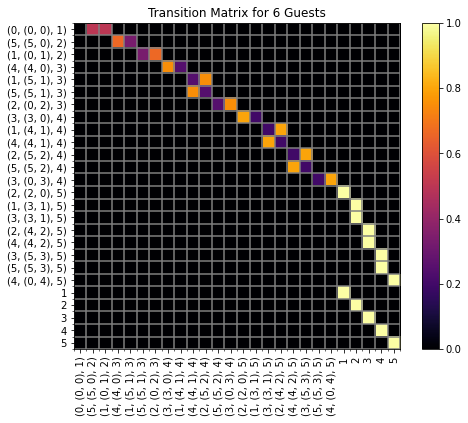In :
# adjacency matrix is the matrix whose i,j is 1 if there is a transition from i to j
# and 0 otherwise

# construct the adjacency graph (for this problem, it's a directed tree)
G = nx.relabel_nodes(G, dict(zip(range(len(G.nodes())), state_labels)))

# Create a dictionary of edge labels
edge_labels = dict()
for i_start, state_start in enumerate(states):
state_label_start = state_labels[i_start]
idx_start = state_to_idx[state_start]

for i_end, state_end in enumerate(states):
state_label_end = state_labels[i_end]
idx_end = state_to_idx[state_end]

# if the graph has an edge, and the nodes are ditinct (i.e. not a terminal node)
# add the probability as the edge label
if P_adjacency[idx_start, idx_end] and idx_start != idx_end:
edge_labels[(state_label_start, state_label_end)] = '{:0.02f}'.format(P[idx_start, idx_end])

# get layout for graph
pos=graphviz_layout(G, prog='dot')

fig, ax = plt.subplots(figsize=(12, 4), facecolor='w')
plt.title("Cranberry Passing States for {} Guests".format(n_guests))

# print the transition probability
edge_text = nx.draw_networkx_edge_labels(G,pos,edge_labels=edge_labels,font_color='grey', font_size = 7.5, ax=ax)
for _,t in edge_text.items():
t.set_rotation('horizontal')

nx.draw(G, pos, with_labels=True, arrows=True, font_size=10, node_color='lightsteelblue', edge_color='grey', ax=ax)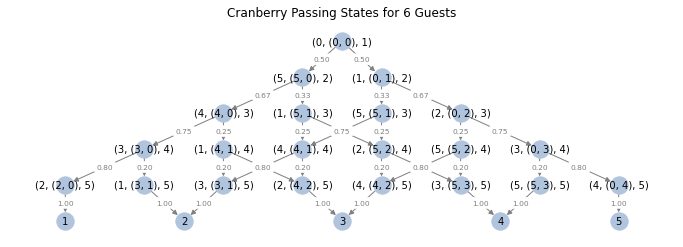In :
def plot_state(state, n_guests, p=(0,0), r=1, ax = None, s=100, s_star=200):
if ax is None:
fig, ax = plt.subplots()

if type(state) == tuple:
k_left, k_right = state
n_served = state

if k_left > k_right:
idxs = [(k_left+i)%n_guests for i in range(n_served)]

else:
idxs = [(k_right-i)%n_guests for i in range(n_served)]

else:
idx_cur = state
idxs = list(range(0, n_guests + 1))

thetas_guest = np.linspace(-np.pi/2, 3*np.pi/2, n_guests + 1)
xs_guest, ys_guest = p + r * np.cos(thetas_guest), p + r * np.sin(thetas_guest)

thetas_table = np.linspace(0, 2*np.pi, 100)
xs_table, ys_table = p + r * np.cos(thetas_table), p + r * np.sin(thetas_table)

ax.plot(xs_table, ys_table, c='k', zorder=100)
ax.scatter(xs_guest, ys_guest, c='lightgrey', edgecolor='k', zorder=101, s = s)

if not type(state) == tuple:
idx_last = state
else:
idx_curr = state
ax.scatter(xs_guest[idx_curr], ys_guest[idx_curr], c='C3', edgecolor='k', zorder=103, s=s)

In :
# plot the adjacency graph
fig, ax = plt.subplots(figsize=(12, 4))
plt.title("Cranberry Passing States for {} Guests".format(n_guests))
pos=graphviz_layout(G, prog='dot')
nx.draw(G, pos, with_labels=False, arrows=True, arrowsize=6, font_size=10, node_size=2, node_color='grey', edge_color='grey')

for state, state_label in zip(states, state_labels):
p = pos[state_label]
plot_state(state, n_guests, p=p, r = 27, s=30, ax=ax)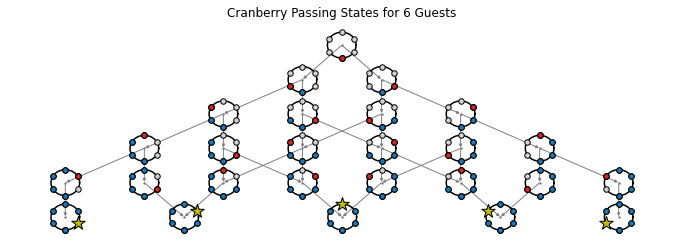In :
p_last = receive_cranberries_last_probabilities(n_guests)
fig, ax = plt.subplots(facecolor='w')
p_last.plot.bar(ax=ax)
ax.get_legend().remove()
ax.set_title('Probability Guest k Receives Cranberry Sauce Last\nNumber of Guests: {}'.format(n_guests))
ax.set_xlabel('Guest Number (k)')

Out:
Text(0.5, 0, 'Guest Number (k)')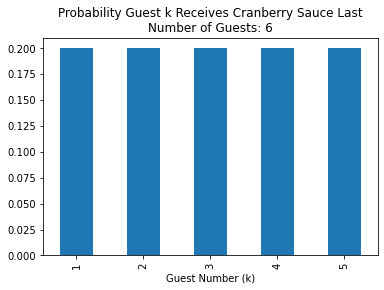## Twenty Guests¶

In :
n_guests = 20
states, state_to_idx, n_states = generate_states(n_guests)
state_labels = [str(s) for s in states]
P = cranberry_passing_transition_matrix(n_guests)

# construct the plot
fig, ax = plt.subplots(figsize = (8,6), facecolor = 'w')
cm = ax.imshow(P,  cmap='inferno')

# major ticks with labels
ax.set_yticks([])
ax.set_xticks([])
ax.set_title("Transition Matrix for {} Guests".format(n_guests))
fig.colorbar(cm);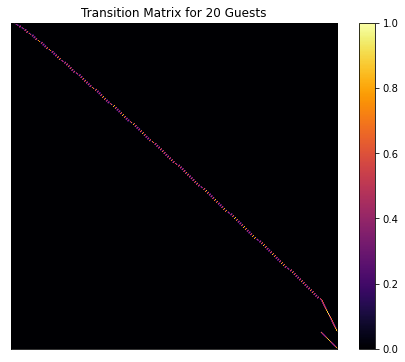In :
# adjacency matrix is the matrix whose i,j is 1 if there is a transition from i to j
# and 0 otherwise

# construct the adjacency graph (for this problem, it's a directed tree)
only_label_terminal = [str(s) if type(s) == int else ('(0,(0,0),1)' if s == (0, (0,0), 1) else ' ') for s in states]
labels = dict(zip(range(len(G.nodes())), only_label_terminal))

fig, ax = plt.subplots(figsize=(12, 4))
plt.title("Cranberry Passing States for {} Guests".format(n_guests))
pos=graphviz_layout(G, prog='dot')
nx.draw(G, pos, with_labels=False, arrows=True, font_size=10, node_size=4, arrowsize=4,
node_color='steelblue', edge_color='grey')

# manually offset the label positions
for k in labels.keys():
tmp = np.array(pos[k])
if labels[k] == '(0,(0,0),1)':
tmp += np.array([0, 60])
pos[k] = tmp
else:
tmp += np.array([0, -75])
pos[k] = tmp
nx.draw_networkx_labels(G,pos,labels,font_size=10,font_color='k');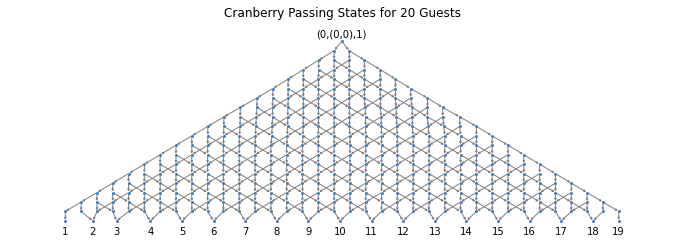In :
p_last = receive_cranberries_last_probabilities(n_guests)
fig, ax = plt.subplots(facecolor='w')
p_last.plot.bar(ax=ax)
ax.set_title('Probability Guest k Receives Cranberry Sauce Last\nNumber of Guests: {}'.format(n_guests))
ax.set_xlabel('Guest Number (k)')
ax.get_legend().remove()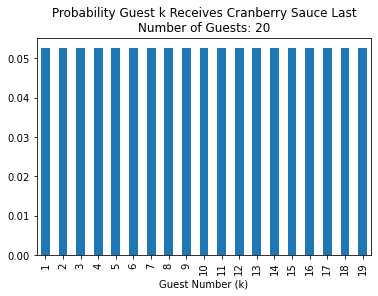## Compute Last Probability and Mean Passes For $n_{guests} = 3,4,...,20$¶

In :
import time

# code isn't set up to run n = 2 since it's trivial
df_p_and_means_all = pd.DataFrame(
[(2, 1, 1, 1)],
columns = ['n_guests', 'guest_number',
'probability_last', 'mean_number_passes']
)

# range to compute
n_guests_lo = 3
n_guests_hi = 20

for n_guests in range(n_guests_lo, n_guests_hi + 1):
tic = time.time()
df_mean_last = mean_passes(n_guests)
toc = time.time()
print("Completed Calculation for n_guests = {} in {:0.03f} seconds".format(n_guests, toc - tic))

# Create a temporary dataframe for current step's results
df_tmp = pd.merge(df_p_last, df_mean_last, left_index=True, right_index=True)
df_tmp['n_guests'] = n_guests
df_tmp = df_tmp.reset_index()

# Concatenate curent step's results on final dataframe
df_p_and_means_all = pd.concat([df_p_and_means_all, df_tmp])

df_p_and_means_all = df_p_and_means_all.reset_index(drop=True)

Completed Calculation for n_guests = 3 in 0.003 seconds
Completed Calculation for n_guests = 4 in 0.003 seconds
Completed Calculation for n_guests = 5 in 0.003 seconds
Completed Calculation for n_guests = 6 in 0.004 seconds
Completed Calculation for n_guests = 7 in 0.007 seconds
Completed Calculation for n_guests = 8 in 0.008 seconds
Completed Calculation for n_guests = 9 in 0.012 seconds
Completed Calculation for n_guests = 10 in 0.021 seconds
Completed Calculation for n_guests = 11 in 0.042 seconds
Completed Calculation for n_guests = 12 in 0.086 seconds
Completed Calculation for n_guests = 13 in 0.178 seconds
Completed Calculation for n_guests = 14 in 0.386 seconds
Completed Calculation for n_guests = 15 in 0.799 seconds
Completed Calculation for n_guests = 16 in 1.757 seconds
Completed Calculation for n_guests = 17 in 3.680 seconds
Completed Calculation for n_guests = 18 in 7.924 seconds
Completed Calculation for n_guests = 19 in 17.812 seconds
Completed Calculation for n_guests = 20 in 40.032 seconds

In :
df_p_and_means_all.to_csv("./output/analytical_prob_and_mean_passes_last.csv", index=False)

In :
# create dataframe for mean number of passes - without conditioning
df_tmp = df_p_and_means_all.copy()

# temporary column for computing unconditional expectation
df_tmp['TMP'] = df_tmp['probability_last'] * df_tmp['mean_number_passes']
df_mean_passes = df_tmp.groupby('n_guests')[['TMP']].sum()['TMP'].reset_index()
df_mean_passes = df_mean_passes.rename(columns = {'TMP' : 'mean_number_passes'})
df_mean_passes['inefficiency'] = (
df_mean_passes['mean_number_passes'] / (df_mean_passes['n_guests'] - 1) - 1
)

# export to file
df_mean_passes.to_csv('./output/analytical_mean_passes_and_inefficency.csv', index=False)

In :
# plot unconditional mean passes
fig, ax = plt.subplots(facecolor='w')
df_mean_passes.plot.bar(x = 'n_guests', y='mean_number_passes', ax=ax)
ax.set_title('Average Number of Passes vs Number of Guests')
ax.set_xlabel('Number of Guests')
ax.set_ylabel('Average Number of Passes')
ax.get_legend().remove()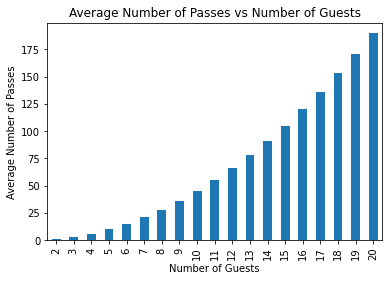In :
# plot inefficiency %
fig, ax = plt.subplots(facecolor='w')
df_mean_passes.plot.bar(x = 'n_guests', y = 'inefficiency', ax=ax)
ax.set_title("Cranberry Passing Inefficiency % vs Number of Guests")
ax.set_xlabel("Number of Guests")
ax.set_ylabel("Inefficiency (%)")
vals = ax.get_yticks()
ax.yaxis.set_major_formatter(mtick.PercentFormatter(1))
ax.get_legend().remove()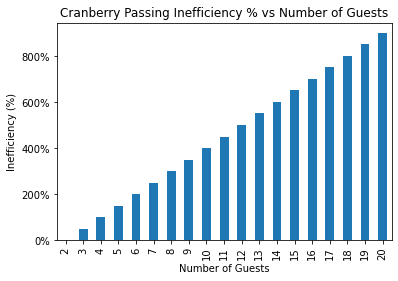In :
# Plot conditional mean number of passes when n_guests = 6
fig, ax = plt.subplots(facecolor='w')
df_p_and_means = df_p_and_means_all[df_p_and_means_all['n_guests'] == 6]
df_p_and_means.plot.bar(x = 'guest_number', y = 'mean_number_passes', ax =ax)

ax.set_title("Average Number of Passes When Guest $k$ is Last\n (Number of Guests = 6)")
ax.set_xlabel("Guest Number $(k)$")
ax.set_ylabel("Average Number of Passes")
ax.get_legend().remove()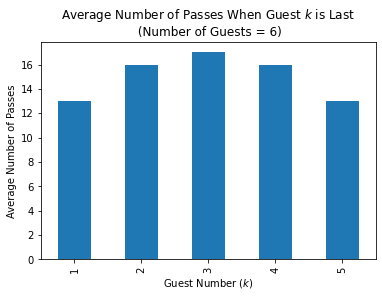In :
# Plot conditional mean number of passes when n_guests = 20
fig, ax = plt.subplots(facecolor='w')
df_p_and_means = df_p_and_means_all[df_p_and_means_all['n_guests'] == 20]
df_p_and_means.plot.bar(x = 'guest_number', y = 'mean_number_passes', ax =ax)

ax.set_title("Average Number of Passes When Guest $k$ is Last\n (Number of Guests = 20)")
ax.set_xlabel("Guest Number $(k)$")
ax.set_ylabel("Average Number of Passes")
ax.get_legend().remove()